# Linear Algebra and Higher Dimensions

Nowadays, governments all try to maximize their GDPs. But if you read Estève’s article on the Human Development Index, you’ll realize that GDP is not all that matters. Too often people and organizations are judged on a single measure that makes them lose sight of the diversity of the goals they must achieved. Other examples include the Shanghai ranking for universities (which led to huge merging projects in France!), calories in diets or “good and evil“. Here’s one last example explained by Barney Stinson on How I Met Your Mother, about how hotness must not be the only criterion to judge a woman’s attractiveness:

I’m going to use Barney’s graph all along, and I hope you won’t get offended. The point of the hot-crazy scale is just to make the article more fun! Also, note that in Barney’s example, Vicky Mendoza’s trajectory should actually rather be a sort of staircase going up…

In all these examples, mathematicians would say that a multidimensional space gets projected on a single dimension, as we turn vectors into scalars.

What??? The multi-what?

Hehe… Let’s enter the breathtaking world of linear algebra to see what I mean!

## Vectors and Scalars

In linear algebra, a vector is fancy word to talk about all the dimensions at once. For instance, according to Barney Stinson, a girl is a combination of craziness and hotness. Now, I know I’m not supposed to do that, but we can give values to these dimensions, ranging from 0 to 10. So, for instance, a girl both not crazy and hot would be 2-crazy and 8-hot. This information is compactly denoted (2,8). Each of the numbers is then called a scalar.

So, a vector is just 2 scalars?

Not necessarily 2! A vector can be made of more scalars. For instance, we may be interested to know if the girl cooks well. And, once again, we can give a value between 0 and 10 describing how well she cooks. In this setting, 3 scalars are necessary. A girl may then be 2-crazy, 8-hot and 6-cooking girl, which we would denote (2,8,6). More generally, the number of scalars we need to describe a girl is called the dimension of the set of girls.

The dimension? Like space dimensions?

Weirdly enough, yes. Exactly like space dimensions. This is something that was remarkably noticed by Frenchman René Descartes in the 1600s, as he unified the two major branches of mathematics of that time, geometry and algebra.

What do you mean? What are you talking about?

Descartes noticed that a single scalar can be associated to a point on an infinite line. And, just like Barney did it, a 2-scalar vector can be placed on a 2-dimensional graph. And, as you’ve guessed, a 3-scalar vector can be placed in our 3-dimension space. Thus, basic geometry can be boiled down to scalars! This is what’s displayed below: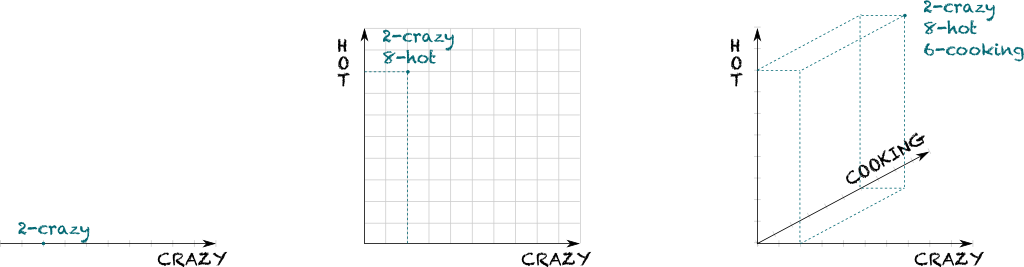So linear algebra is just about studying several scalars all at once rather than a single one?

Basically, yes. But there’s so much more…

## Coordinates

Let’s get back to Barney’s crazy-hot scale. Vectors here are made of two scalars, one for craziness, the other for hotness. But as Barney pointed it out, what rather matters is the location of a girl with regards to the Vicky-Mendoza diagonal. To quote Barney, “a girl is allowed to be crazy as long as she is equally hot”. Now, what’s disappointing with the measures of hotness and craziness is that we need to know both to find out whether a girl is attractive or not. So, let’s introduce two other measures we call attractiveness and extremeness.

How do you define these?

Well, according to Barney, the more a girl is above the Vicky-Mendoza diagonal, the more attractive she is. Thus, attractiveness can be defined as how far away from the diagonal a girl is. Meanwhile, a girl is extreme if she’s near the top right corner. Let’s illustrate with figures: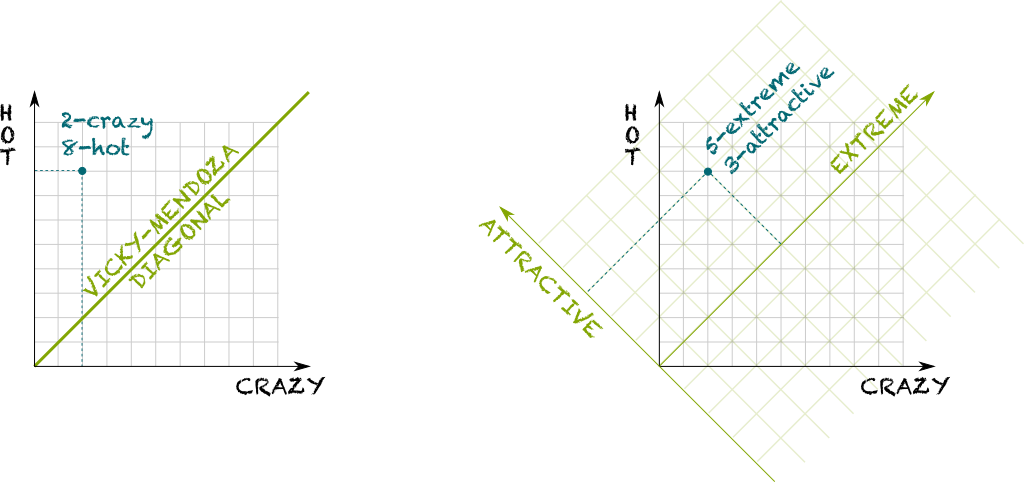By now using the extreme-attractive scale, a 2-crazy and 8-hot girl can be described as a 5-extreme and 3-attractive girl. In more mathematical terms, we say that this girl has coordinates (2,8) on the crazy-hot scale, and (5,3) on the extreme-attractive scale. Now here’s the tricky part of linear algebra: While a girl can be defined as 5-extreme and 3-attractive, it makes no sense to only say that she is 3-attractive!

What?

More precisely, the attractiveness of a girl can only be defined within a coordinate system. So, you can talk about her attractiveness in the extreme-attractiveness scale, but you cannot define attractiveness alone! And this holds for craziness, hotness and extremeness as well!

What the hell? Why?

Let’s consider our 2-crazy and 8-hot girl, and let’s look at her coordinates in the hot-attractive scale.

Aren’t these (8,3)?

Well, let’s draw a graph to figure it out!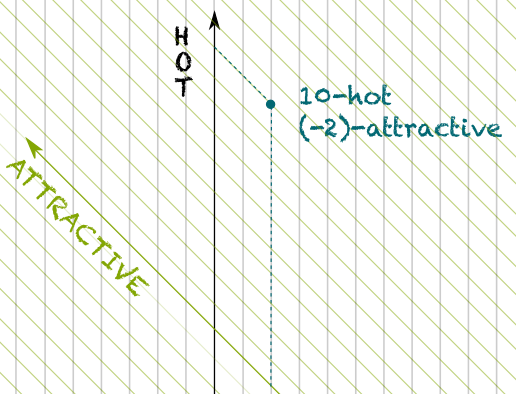As you can see, on a hot-attractive scale, the coordinates of the girl are not (8,3). They are (10,-2). In particular, the attractiveness is now… negative!

I’m completely lost here… What the hell is happening?

In essence, what we see here is that the concept of coordinates strongly depend on the scales we choose. These scales are called coordinate systems, or basis. But, more importantly, what we have shown here is that there’s nothing fundamental in the concept of coordinates. Any modification of the axes change these coordinates. Another phrasing of this remark consists in saying choosing a first and a second dimension is completely artificial and not fundamental. This is what’s brilliantly explained by Henry Reich on Minute Physics:

To understand what Henry means by “different kinds of dimension of spacetime”, check my articles on special and general relativity. Beware, this is trickier than it sounds!

To fully understand what’s going on here, we actually need to study spaces independently from a coordinate system. That’s where linear algebra becomes awesome!

The key concept for a geometrical description of linear algebra is to think of vectors as motions. Such motions can typically be represented by arrows. For instance, the following figure displays the motion of Barney’s face from bottom left to top right: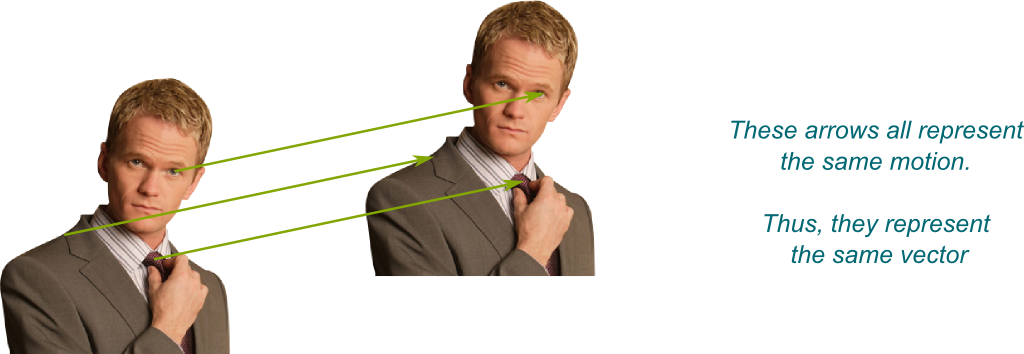Crucially, the arrows of the figure all represent the same motion. And since a vector is a motion (not an arrow!), they all represent the same vector. In other words, you can move an arrow wherever you want, it will still stand for the same vector.

But what’s the point in considering vectors as motions?

We’ll now get to do some cool algebra of vectors, independently from any basis! At its core, algebra is the study of operations. And there are two very natural operations we can do with motions. First, we can combine motions. This is called addition. Here’s how it’s done: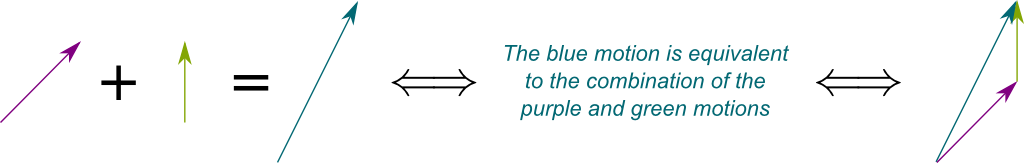The reason why we define this combination of motions as an addition is because it has strong similarities with the classical addition of numbers. Namely, the addition of vectors yields a zero element (the motion which consists in not moving!), is commutative and associative (the order in which we combine several motions does not matter) and can be inverted (by the opposite motion). In pure algebra terms, this means that a vector space is a commutative group.

Another important operation which can be done with a motion is its rescaling. Algebraically, this stretching corresponds to multiplying the motion by a scalar. For instance, we can double a motion (multiplication by 2), or invert it (multiplication by -1). This operation is known as scalar multiplication.

More precisely, the set of scalars must be a field, which means that an addition, subtraction, multiplication and division (except by zero) must all be well-defined. In practice, this set of scalars is often one of the following sets: $\mathbb Q$, $\mathbb R$, $\mathbb C$, $\mathbb Z/p\mathbb Z$. If the set of scalar is only a ring (which means division is not defined in general) like $\mathbb Z$, then we talk about modules rather than vector spaces.

So, in essence, a vector space is simply a set of vectors which can be added and scalar-multiplied. In fact, everything in linear algebra has to be based on merely additions and scalar-multiplications!

Really? Even coordinate systems?

Yes! So far, a basis has been defined by two graduated axes. But we should rather think of bases in terms of motions too!

Really? How?

Let’s get back to the crazy-hot scale. In this setting, we can define the crazy unit vector as the motion of 1 towards craziness, and the hot unit vector as the motion of 1 towards hotness.

OK… So scales are defined by the unit vectors, but this doesn’t say much about coordinates!

There’s one last ingredient we need for this: The origin. A girl can now be located by the motion from the origin to her location in the crazy-hot scale. And, amazingly, this motion can be decomposed by certain amounts of unit motions towards craziness and towards hotness. For instance, a 2-crazy and 8-hot girl can be described as a motion from the origin by 2 unit vectors of craziness and 8 unit vectors of hotness! This is what’s pictured below: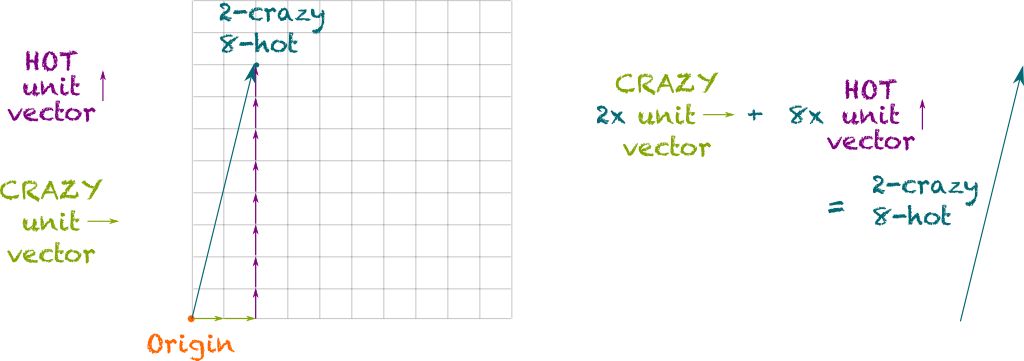In fact, all girls are associated to vectors which can be decomposed as a sum of the crazy and hot unit vectors! And this holds for other bases too: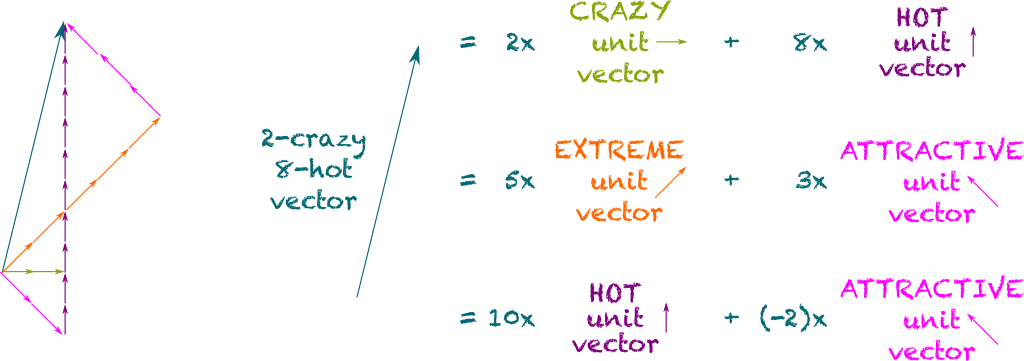So, what’s really meant by coordinates in a coordinate system is how a vector can be decomposed into a sum of the unit vectors of the basis, right?

Yes! And now, this definition doesn’t require an a priori on the coordinate system! It’s solely based on additions and scalar multiplications! Isn’t it amazing?

Hummm… I guess…

OK, let me show you how else algebra can blow your mind now then! So far, to decompose the 2-crazy and 8-hot vector into other bases, I had to draw the figure on the left. This technique is great for our intuition, but it’s not very fast…

Are you going to show how to decompose a vector quickly in any basis?

Yes! For instance, let’s decompose the 2-crazy and 8-hot vector in the extreme-attractive scale without geometry! The key is to notice that a crazy vector is half an extreme unit vector minus half an attractive unit vector. Meanwhile, a hot unit vector is itself a sum of half an extreme unit vector and half an attractive unit vector. Let’s replacing them in our first equality above and involve the power of algebra: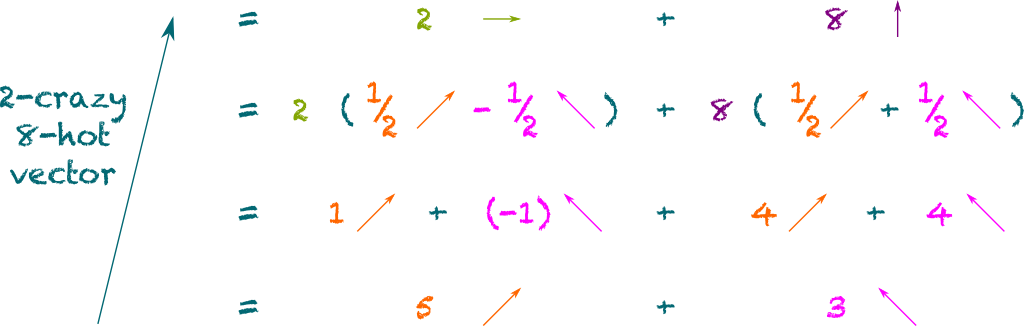As often done in algebra, for the simplicity of formulas, I’ve removed all signs of scalar multiplications.
Can such a decomposition as a sum of unit vectors always be done?

No. The family of unit vectors must satisfy 2 criteria. First, the decomposition must exist, in which case we say that the unit vectors are spanning. And second, the decomposition must be unique. When this is the case, the family is called linearly independent. When the family of unit vectors is both spanning and linearly independent, it forms a basis and defines a proper coordinate systems.

Formally, a family $(e_1, …, e_n)$ of vectors is spanning if, for any vector $x$, there exist scalar coordinates $(\lambda_1, …, \lambda_n)$ such that $x = \lambda_1 e_1 + … + \lambda_n e_n$. A family $(e_1, …, e_n)$ is linearly independent if the only coordinates $(\lambda_1, …, \lambda_n)$ such that $\lambda_1 e_1 + … + \lambda_n e_n = 0$ are the zero coordinates $(0, …, 0)$.

Amazingly, for a given set of vectors, the number of vectors in a basis is always the same. This invariant is called the dimension of the vector space. Typically, the crazy-hot scale is of dimension 2 because any coordinate system requires 2 scalars. But, as we have seen, the crazy-hot-cooking scale has dimension 3, and we can easily go on defining higher dimension vector spaces! In fact, dimensions can even be infinite!

Really?

Yes! That’s the case of infinite sequences. Indeed, these form a vector space, as the addition of two infinite sequences (add terms by terms!) and the scalar multiplication by a scalar (multiply all terms by the scalar) are naturally defined. Yet, there is no way of decomposing all sequences into a basis made of a finite number of unit vectors!

We can still guarantee that infinite dimension spaces have bases. In this case, a basis is a family of vectors such that any vector $v$ can be decomposed as a finite sum of basis vectors. BUT, weirdly enough, proving the existence of these bases requires the very controversial and not-always-accepted axiom of choice, as the proof involves considering minimal spanning family and maximal linearly independent ones. In fact, bases of infinite sequences are never explicit!

## Matrices

As you’ve probably guessed it, the method we used to perform algebraically a basis change was perfectly generic. So let’s really generalize it! To do so, instead of studying the 2-crazy and 8-hot girl, we’ll focus on all girls at once!

Can we do that?

Yes! Let’s just call them $x$. More precisely, all girls can be described as $x_{crazy}$-crazy and $x_{hot}$-hot. And, as we do the exact same manipulation as earlier, we obtain the following formulas: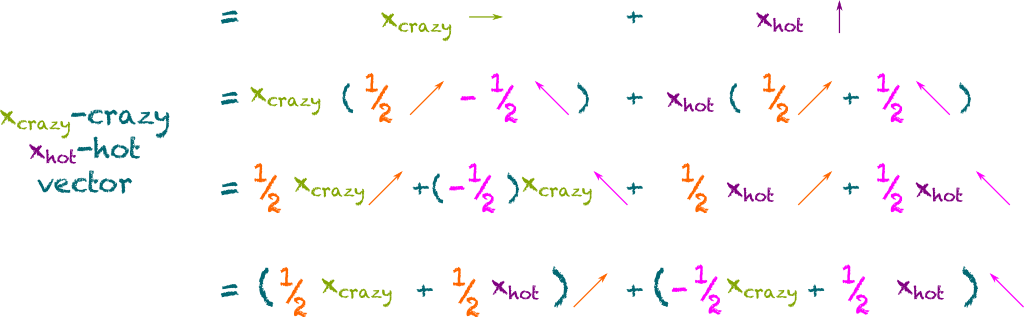Hummm… OK… What now?

Now, we know that the terms before the unit vectors are the coordinates! Thus, we can write the coordinates of a girl in the extreme-attractive scales as a function of their coordinates in the crazy-hot scale. Here’s what we get: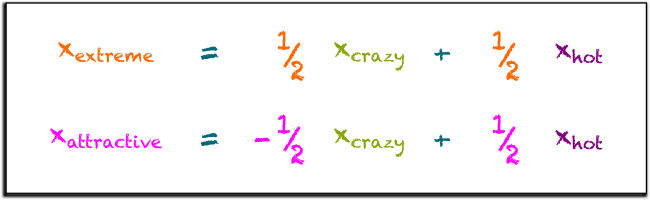As you can see, each coordinate in the extreme-attractive scale is actually a simple sum of the coordinates in crazy-hot scales multiplied by constant coefficients. This means that the relation between the two scales is perfectly described by these coefficients. To keep track of these coefficients, mathematicians have decided to put them in tables, called matrices.

Matrices? Like the movie matrix?

Exactly! Although, I’m not sure why they named the movie like that…

Basically, a matrix is just a table. But, interestingly, there are plenty of operations which can be done with matrices. In particular, we can do addition, scalar multiplication (which means matrices are vectors!), and multiplication (which means that they are more than just vectors!). These operations are beautifully described in Bill Shillito in the great TedEd video:

Careful though, matrix multiplication is not commutative, which means that $MN \neq NM$. In fact, if matrices $M$ and $N$ aren’t of the right size, it might be that $MN$ is well-defined but $NM$ not.

Let’s apply that to basis change! By arranging the coefficients of the formulas we got in a natural 2-lines and 2-columns matrix, and the 2-scalar coordinates in 2×1 matrices, we get the following equality: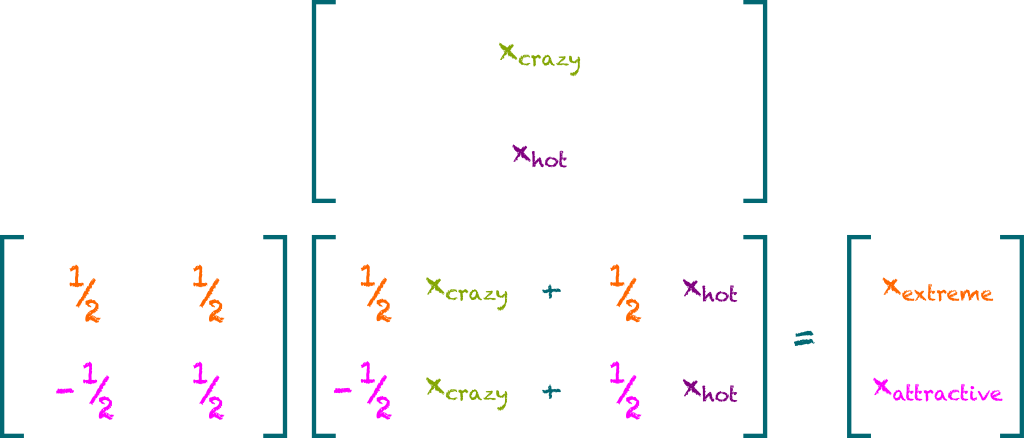Pretty cool, isn’t it?

Hummm… So, when we do linear algebra, we always have to write huge matrices?

Hehe… That’s where we use the full potential of algebra! A huge matrix can be referred to by any letter you want! So, for instance, let’s call $P_{CH}^{EA}$ our 2×2 matrix, $X_{CH}$ the 2×1 matrix of crazy-hot coordinates and $X_{EA}$ the 2×1 matrix of the extreme-attractive coordinates, the formula above is simply denoted $X_{EA} = P_{CH}^{EA} X_{CH}$. So, once you know $P_{CH}^{EA}$, a mere matrix multiplication yields the basis change! Now, this matrix multiplication may still sound hard to compute, but don’t forget we have computers to do that now!

Well, you still need to compute $P_{CH}^{EA}$…

Haven’t you noticed what $P_{CH}^{EA}$ stood for? The two columns are in fact the coordinates of the crazy and hot unit vectors in the extreme-attractive scale! More generally, the coordinates of the former basis in the latter one are the only information needed to compute $P_{CH}^{EA}$! Isn’t that awesomely simple?

I wouldn’t say that… But it’s pretty cool indeed!

Let’s now generalize matrix multiplications.

## Linear Transformation

Once we’ve chosen a basis of a vector space, all vectors can be represented by their coordinates, which can be gathered in a column matrix $X$, simply called column. Operations can then be done to that column, like the multiplication $MX$ by a matrix $M$. We then obtain a new column $Y = MX$, whose coordinates in turns represent some vector in the chosen basis. Now, that kind of going back-and-forth between vectors and their corresponding columns is not something I appreciate…

Why?

Because all this reasoning pre-assumes we had bases to work on. This may not be the case for some vector spaces. And even if these do yield bases, it may not be natural to work with them! So, what mathematicians have done is taking out the essence of the operations of multiplying by $M$: linearity.

Linearity?

After all, we’re doing linear algebra, right?

I’ve read that, but I’m still not sure what that means!

Linearity means that everything is made of additions and scalar multiplications. And everything is compatible with these. Crucially, taking a column $X$ and transforming it into $MX$ is a linear operation. This means that it is compatible with addition and scalar multiplication, which, formally, corresponds to identities $M(X+Y) = MX + MY$ and $M(\lambda X) = \lambda (MX)$ where $\lambda$ is a scalar. But, once again, I don’t want to study columns… I want to study vectors!

So you’re going to define linear transformations of vectors, right?

Precisely. Let’s denote $x$ any vector, and let’s transform it into another vector we call $u(x)$. Then, $u$ is a linear transformations of vectors if $u(x+y) = u(x) + u(y)$ and $u(\lambda x) = \lambda u(x)$.

Can you give an example?

Sure! A girl in the crazy-hot scale is some vector $x$. We can transform this vector into the column of its coordinates in the crazy-hot scale. By decomposing all vectors as a sum of crazy and hot unit vectors, you can show that this transformation is indeed linear. Another example is transforming $x$ into its crazy coordinate in the crazy-hot scale. This time, $u(x)$ would be a scalar, and, once again, you can show that $u$ is linear. In fact, this is an example of the projections I was referring to in the introduction!

Are linear transformations always about retrieving coordinates?

No! Other geometrical examples are homothety (multiply all vectors by a constant) and rotation, you can read about in my articles on symmetries and complex numbers.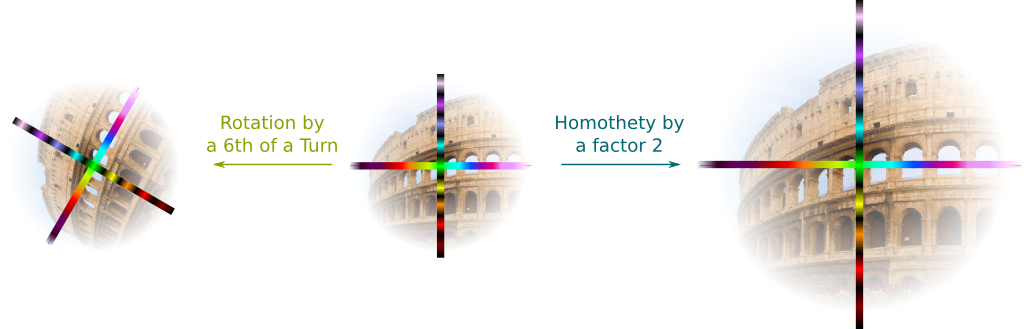One cool fact about linear transformations is that you fully know them once you know how they transform a basis. Indeed, if $(e_1, …, e_n)$ is a basis, any vector $x$ can be decomposed by $x = x_1 e_1 + … + x_n e_n$. By linearity, we then have $u(x) = x_1 u(e_1) + … + x_n u(e_n)$. The linear transformation $u$ is fully represented by the matrix whose columns are the coordinates of the vectors $u(e_1), …, u(e_n)$ in a basis of the output vector space.

Formally, given bases of the input and output vector spaces, the mapping of a linear transformation $u$ to the matrix described above is in fact a fundamental isomorphism of algebra. This means that it is a one-to-one map, which is linear and compatible with the multiplication of matrices, which is associated to the composition of linear functions.

However, even given a corresponding matrix, it’s usually hard to fully understand linear transformations.

Are there other ways?

Yes! Most importantly are the images and the kernels of linear transformations. The image is the range of values $u$ can yield. For instance, if $u$ maps vectors to a column whose entries are all the first coordinate in a certain basis, then $u$ always creates columns with all-identical entries. Meanwhile, the kernel contains all the solutions of equation $u(x) = 0$. This is very important for solving equations like $u(x)=y$, which correspond to linear systems of equations. Then, if $x_0$ is a solution and $x$ is in the kernel, then $x+x_0$ is also solution. Indeed, $u(x_0 + x) = u(x_0) + u(x) = y + 0 = y$.

Images and kernels are in fact vector subspaces. An important theorem of linear algebra then links them. It says that the dimensions of the kernel and of the image add up to the dimension of the input vector space.

Finally, note that the most important kind of linear transformations are those which transform a vector into a vector of the same space. These are called endomorphism. Then, it’s common to use the same basis for inputs and outputs to write the corresponding matrices. Since each column and each row corresponds to a unit vector of the basis, these matrices are then square matrices.

In particular, endomorphisms can be multiplied with one another without restriction. Equivalently, the multiplication is perfectly well-defined for square matrices of a given size. This means that the set of endomorphisms and the set of square matrices are what mathematicians call algebras.

A powerful way to study endomorphisms and square matrices is the study of eigenvectors and eigenvalues. An eigenvector is a vector such that its image is proportional to itself. So typically, $x$ is an eigenvector if $u(x) = \lambda x$. The scalar $\lambda$ is then an eigenvalue of $u$. The study of eigenvalues is especially important to quantum mechanics, where waves stationary for the Schrödinger equation are eigenvectors.

The study of eigenvalues is a very important area of mathematics. If you can, please write about it!

## Let’s Conclude

It is hard to stress how important linear algebra is to mathematics and science. To do so, let me end by listing some of my articles which require linear algebra for a full understanding: linear programming, spacetime of special relativity, spacetime of general relativity, infinite series, model of football games, Fourier analysis, group representation, high-dynamic range, complex numbers, dynamics of the wave function, game theory, evolutionary game theory… In my present research on mechanism design, linear algebra is the essential ground I stand on without even noticing it. That’s how essential linear algebra is!

As I have tried to give you the intuition of linear algebra, it might have sounded long to introduce. Yet, the power of algebra could get you much further much quicker. This is why I strongly recommend you to learn more and become more and more familiar with algebra. Once you do master it better, you’ll be unstoppable in the understanding of complex (linear) systems!

## More on Science4All

The Magic of AlgebraBy Lê Nguyên Hoang | Updated:2016-02 | Views: 3798
The power of algebra lies in abstraction, and abstraction is basically forgetting. By retracing the History of algebra from its roots to more recent advancements, this article unveils the numerous breakthrough in our understanding of the world, by abusing of the power of forgetting.

Colours and DimensionsBy Lê Nguyên Hoang | Updated:2015-12 | Views: 4585
You've probably learned early on that there are three primary colours. But why three? And why these three? Surprisingly, the answer lies in the beautiful mathematics of linear algebra and (high) dimension spaces!

Optimization by Linear ProgrammingBy Lê Nguyên Hoang | Updated:2016-02 | Views: 7722
Operations Research deals with optimizing industrial systems. Those systems can be very complex and their modeling may require the use of hundreds, thousands or even millions of variables. Optimizing over millions of variables may seem impossible, but it can be done if the optimization problem has a linear structure. Learn more on this linear structure and optimization solutions!

Imaginary and Complex NumbersBy Lê Nguyên Hoang | Updated:2016-02 | Views: 6779
My first reaction to imaginary numbers was... What the hell is that? Even now, I have trouble getting my head around these mathematical objects. Fortunately, I have a secret weapon: Geometry! This article proposes constructing complex numbers with a very geometrical and intuitive approach, which is probably very different from what you've learned (or will learn).

The Surprising Flavor of Infinite SeriesBy Lê Nguyên Hoang | Updated:2020-01 | Views: 11232
1+2+4+8+16+...=-1, as proven by Henry Reich on Minute Physics! Now, as a mathematician, I must say that his proof is far from being rigorous. In fact, anyone familiar with the surprising flavor of infinite series should not find it convincing. Surprisingly though, his proof can be rigorously and naturally justified! Find out how!

Spacetime of General RelativityBy Lê Nguyên Hoang | Updated:2016-01 | Views: 13988
Most popular science explanations of the theory of general relativity are very nice-looking. But they are also deeply misleading. This article presents you a more accurate picture of the spacetime envisioned by Albert Einstein.